# Digital Electronics - Combinational Logic Analysis

Exercise : Combinational Logic Analysis - General Questions
1.

Referring to the GAL diagram, which is the correct logic function?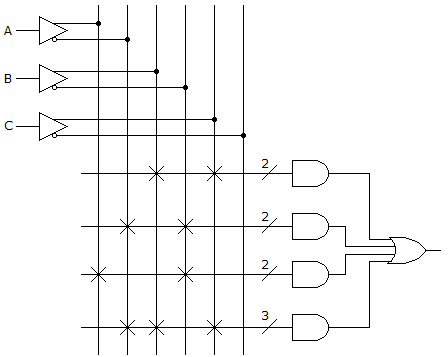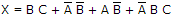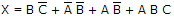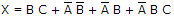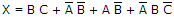Explanation:
No answer description is available. Let's discuss.

2.
The output of an exclusive-NOR gate is 1. Which input combination is correct?
A = 1, B = 0
A = 0, B = 1
A = 0, B = 0
none of the above
Explanation:
No answer description is available. Let's discuss.

3.

The Boolean SOP expression obtained from the truth table below is ________.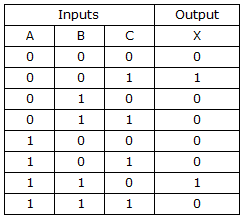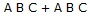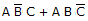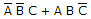None of these
Explanation:
No answer description is available. Let's discuss.

4.
The 8-input XOR circuit shown has an output of Y = 1. Which input combination below (ordered A – H) is correct?10111100
10111000
11100111
00011101
Explanation:
No answer description is available. Let's discuss.

5.

Implementing the expression AB + CDE using NAND logic, we get: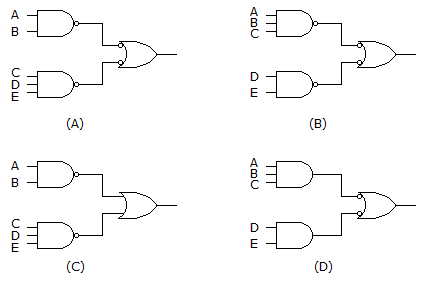(A)
(B)
(C)
(D)